Chapter 4 Part 1 - AD,AS and Related Concepts

Economics Class 12
Macroeconomics

## What is MPC?

MPC means Marginal Propensity to Consume

It is ratio of Change in Consumption to Change in Income

MPC = Change in Consumption/Change in Income

= Delta C /Delta Y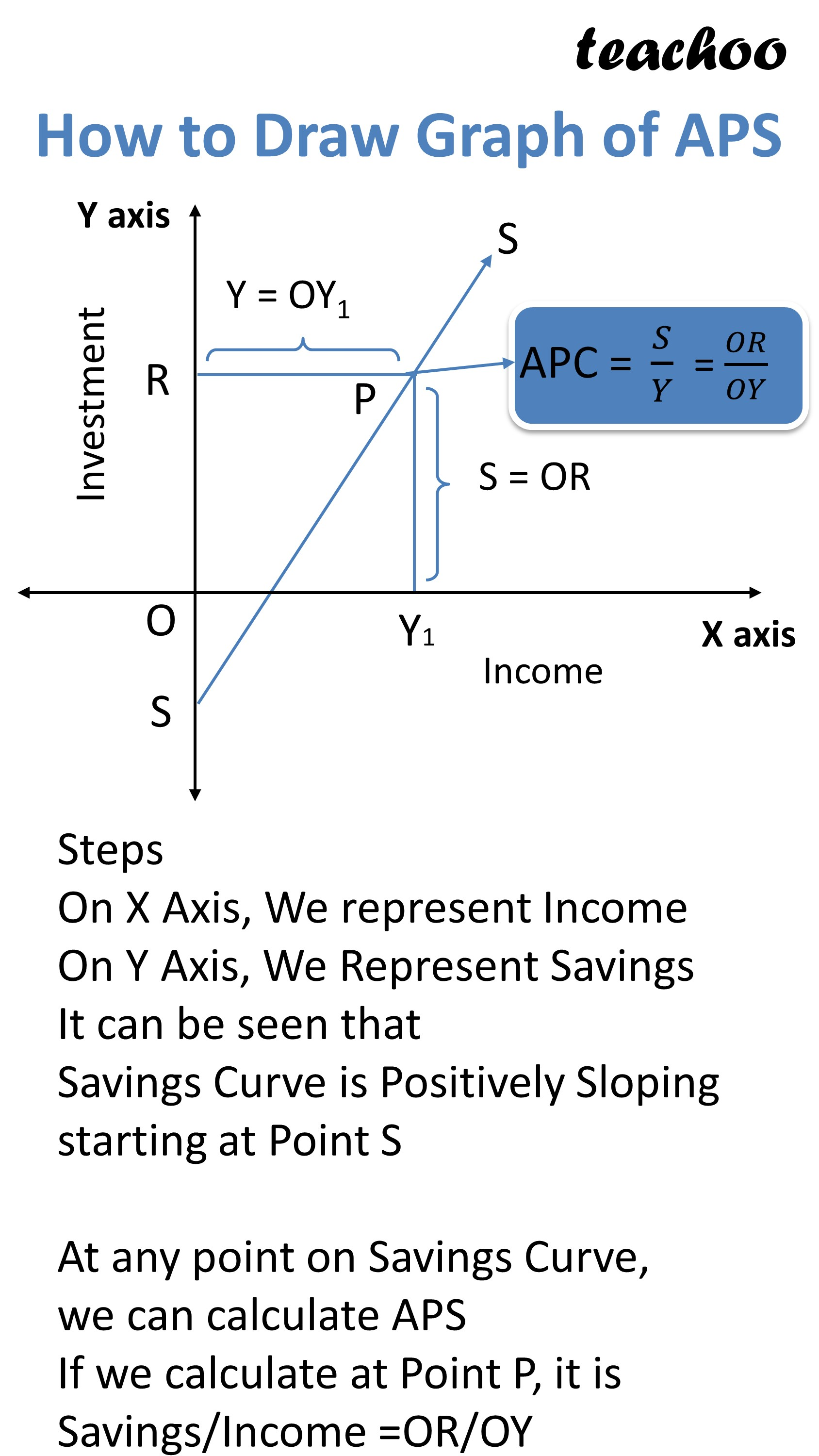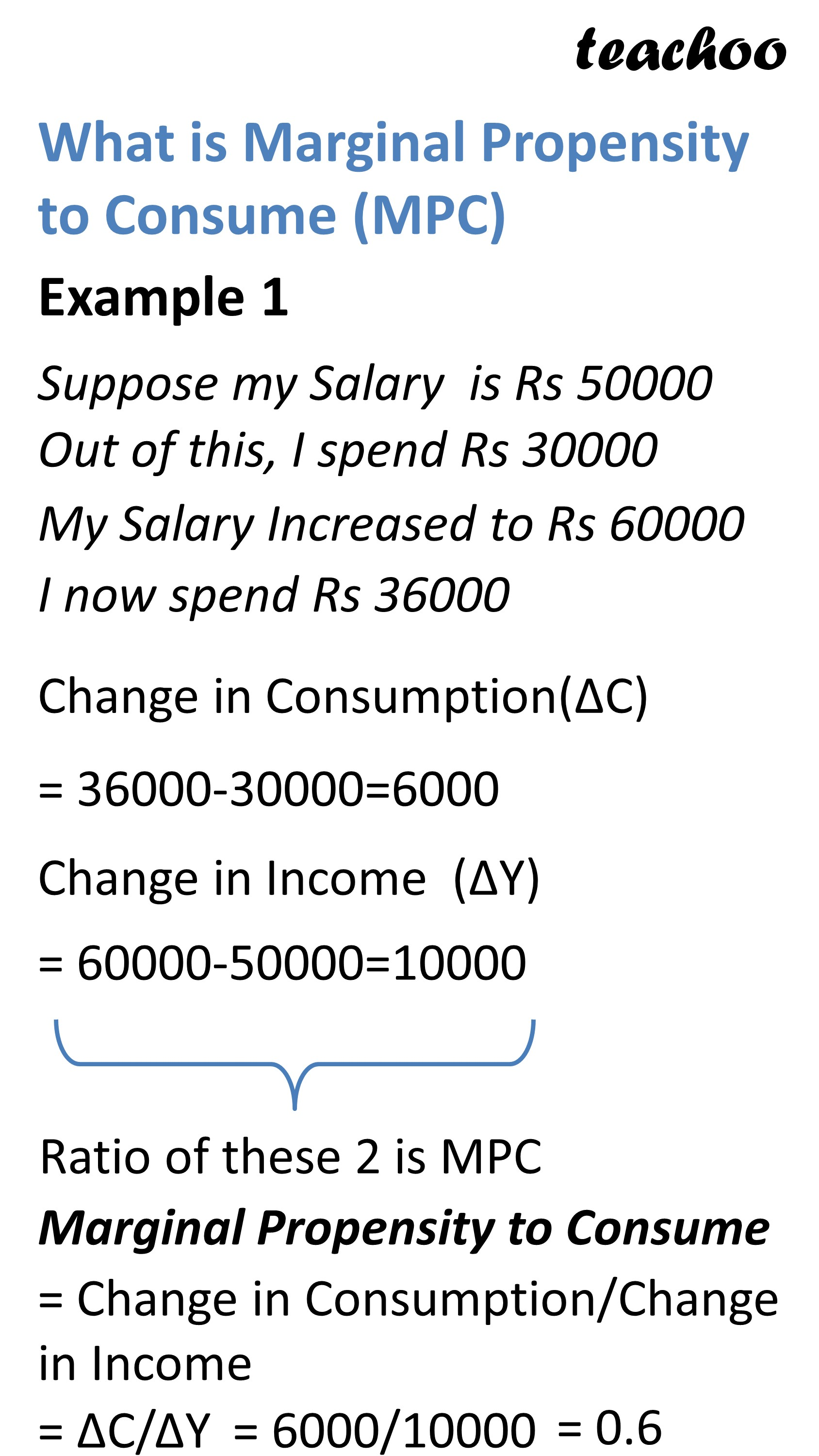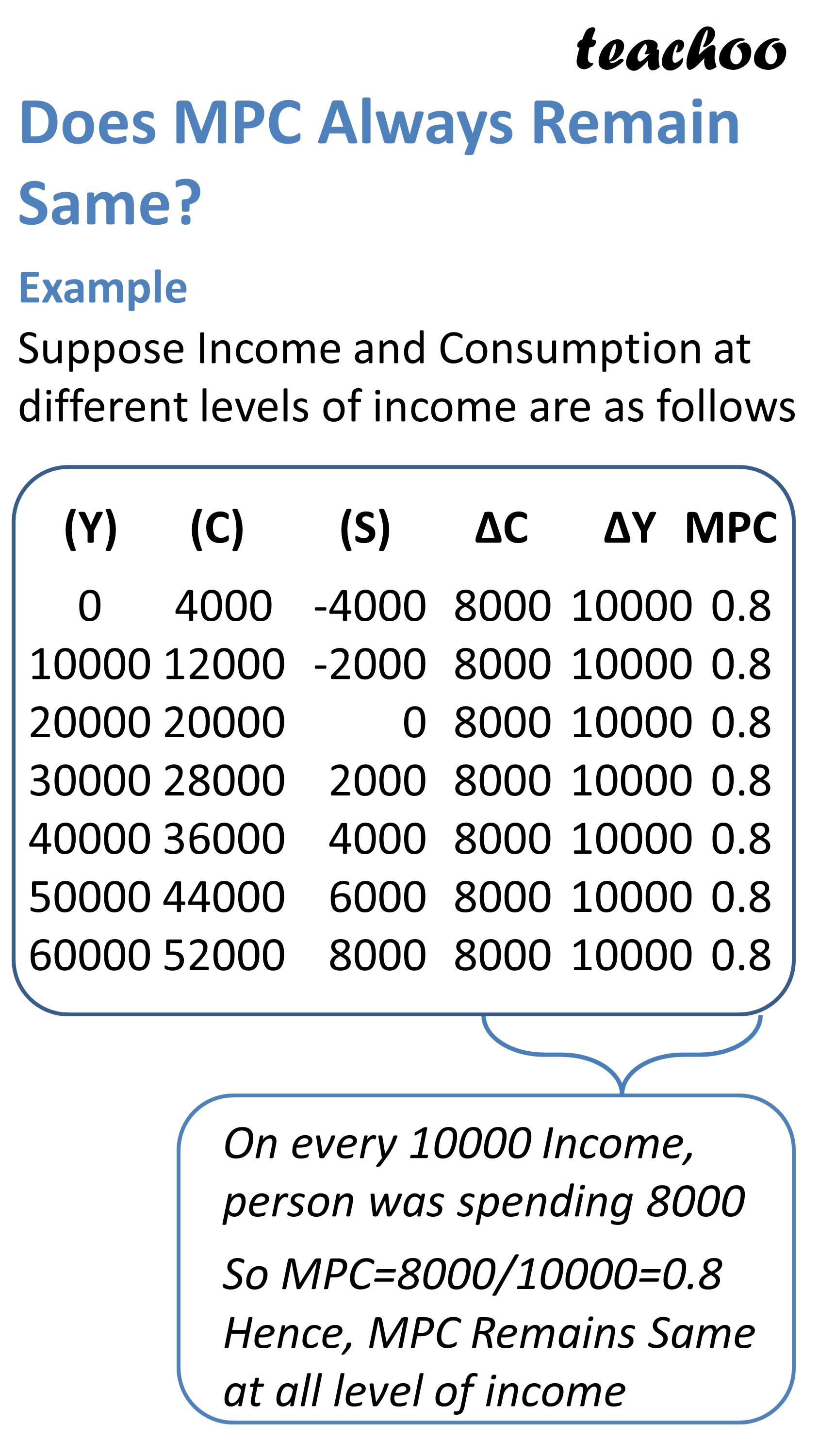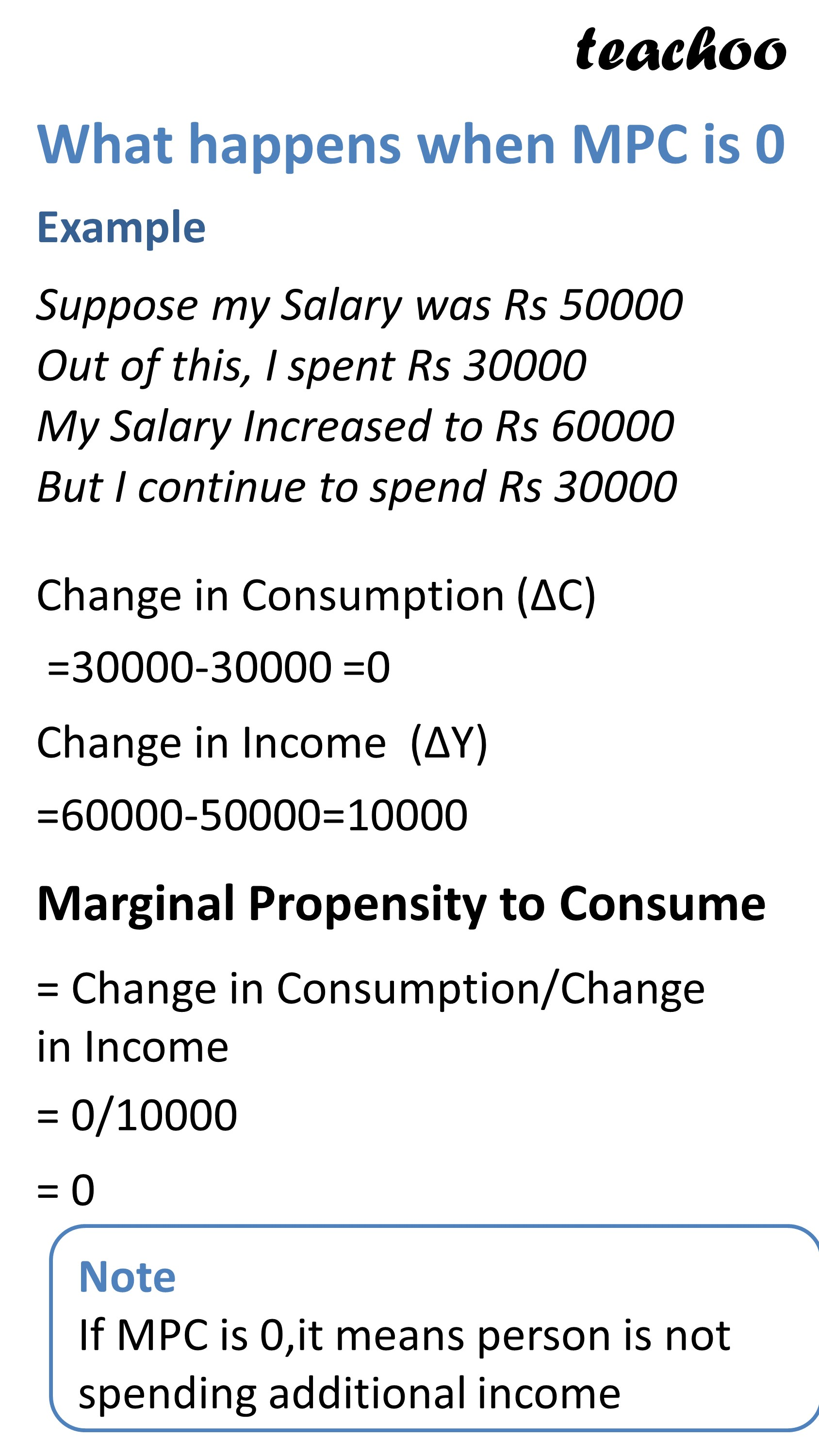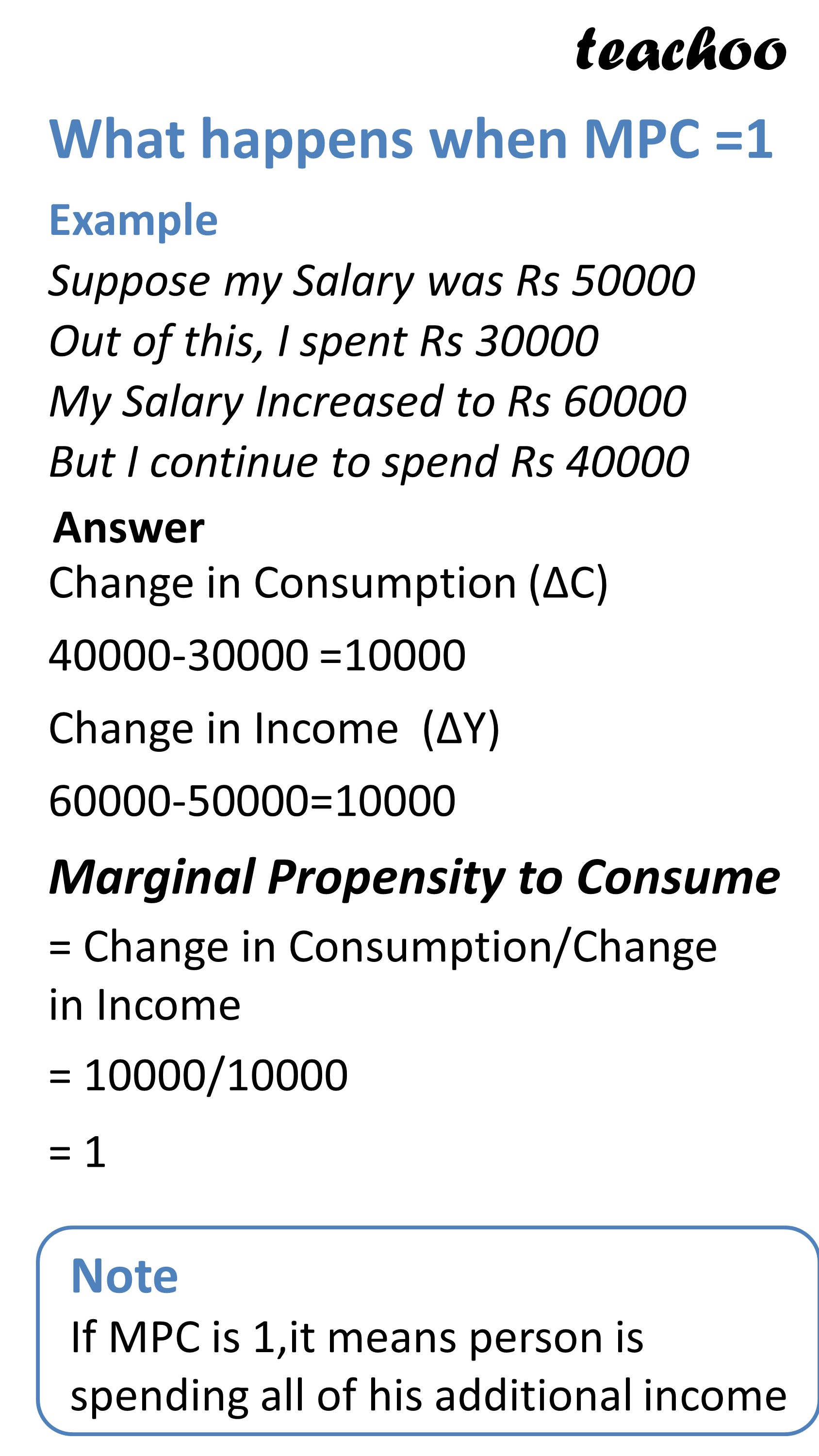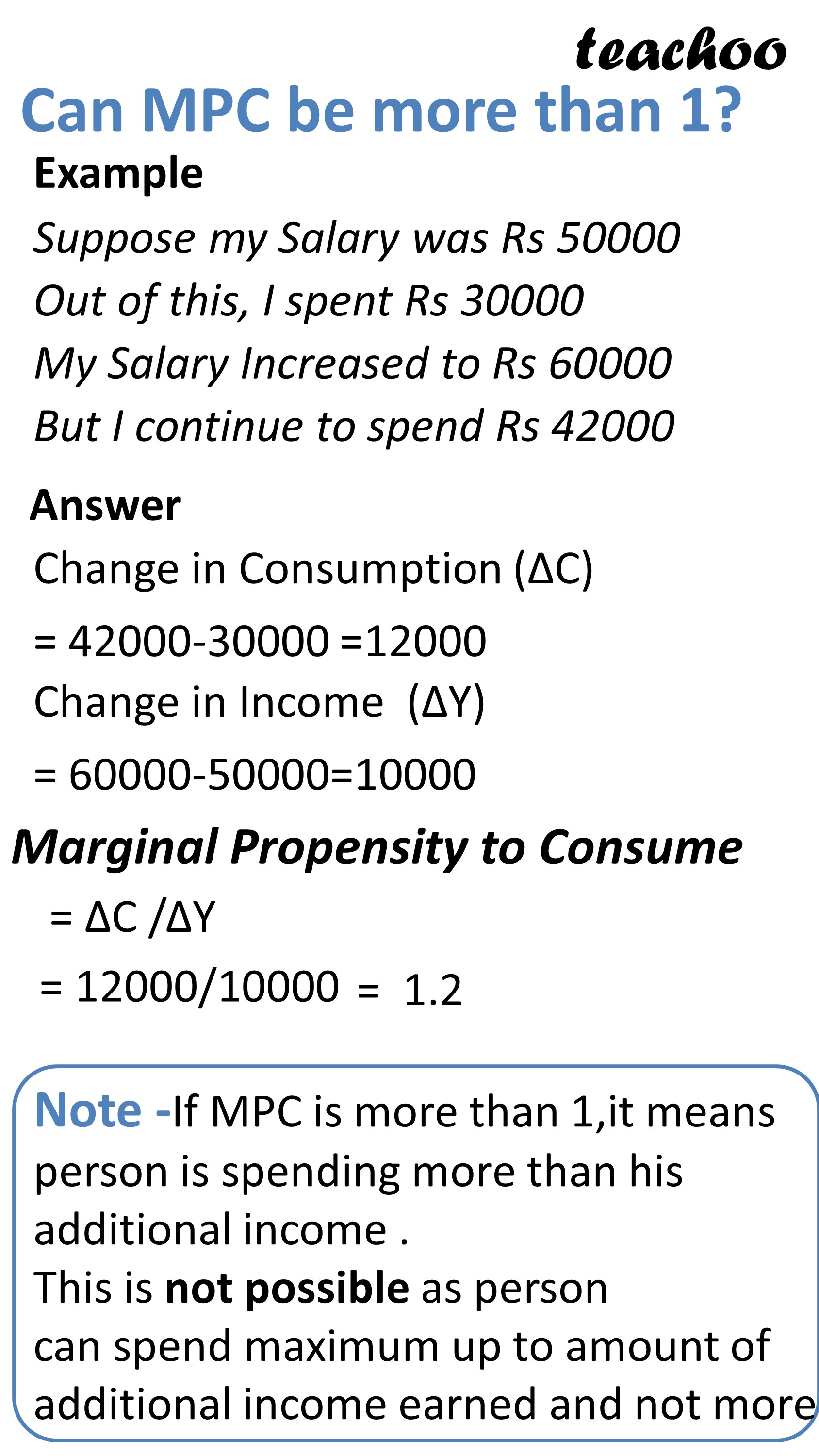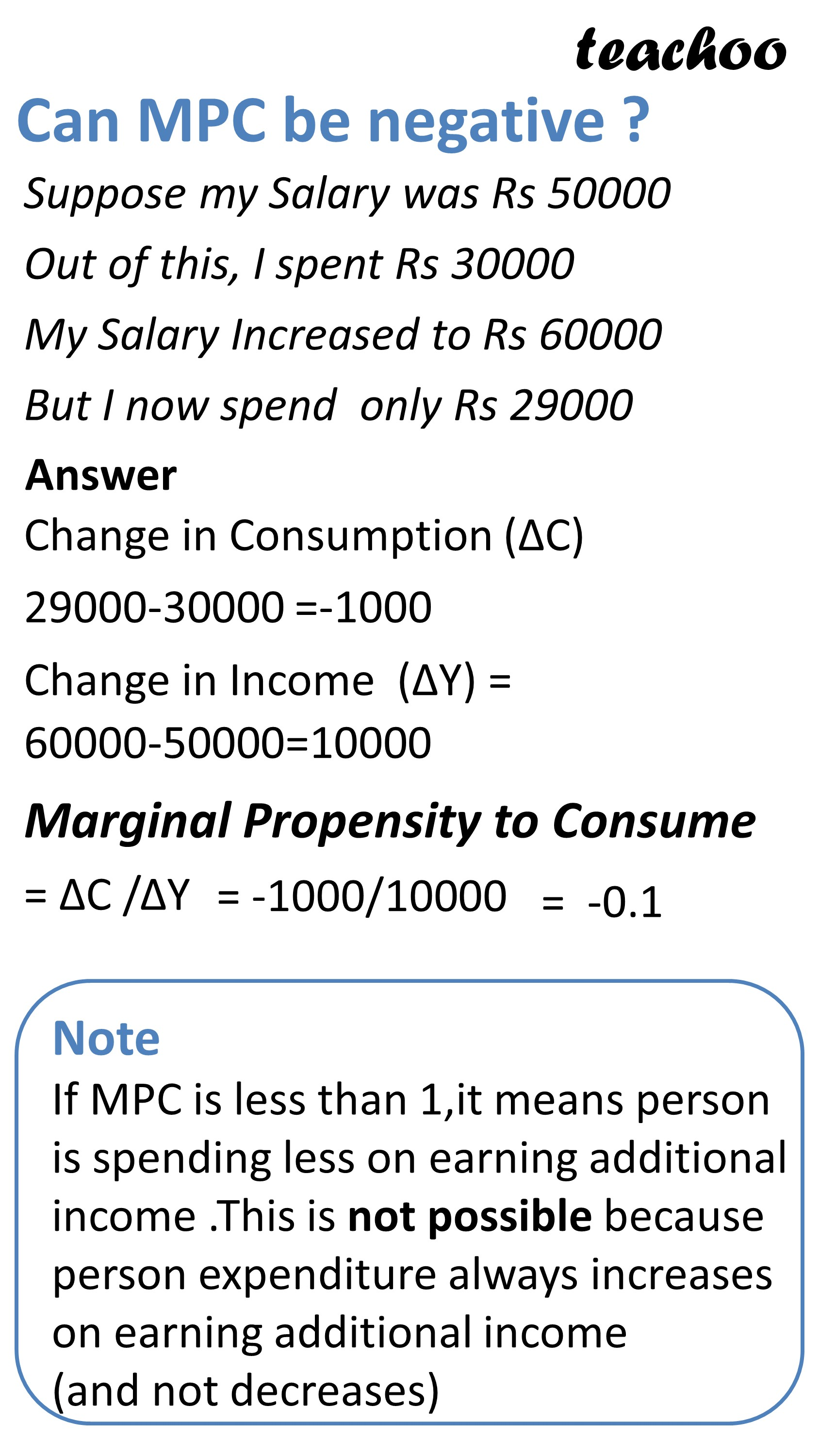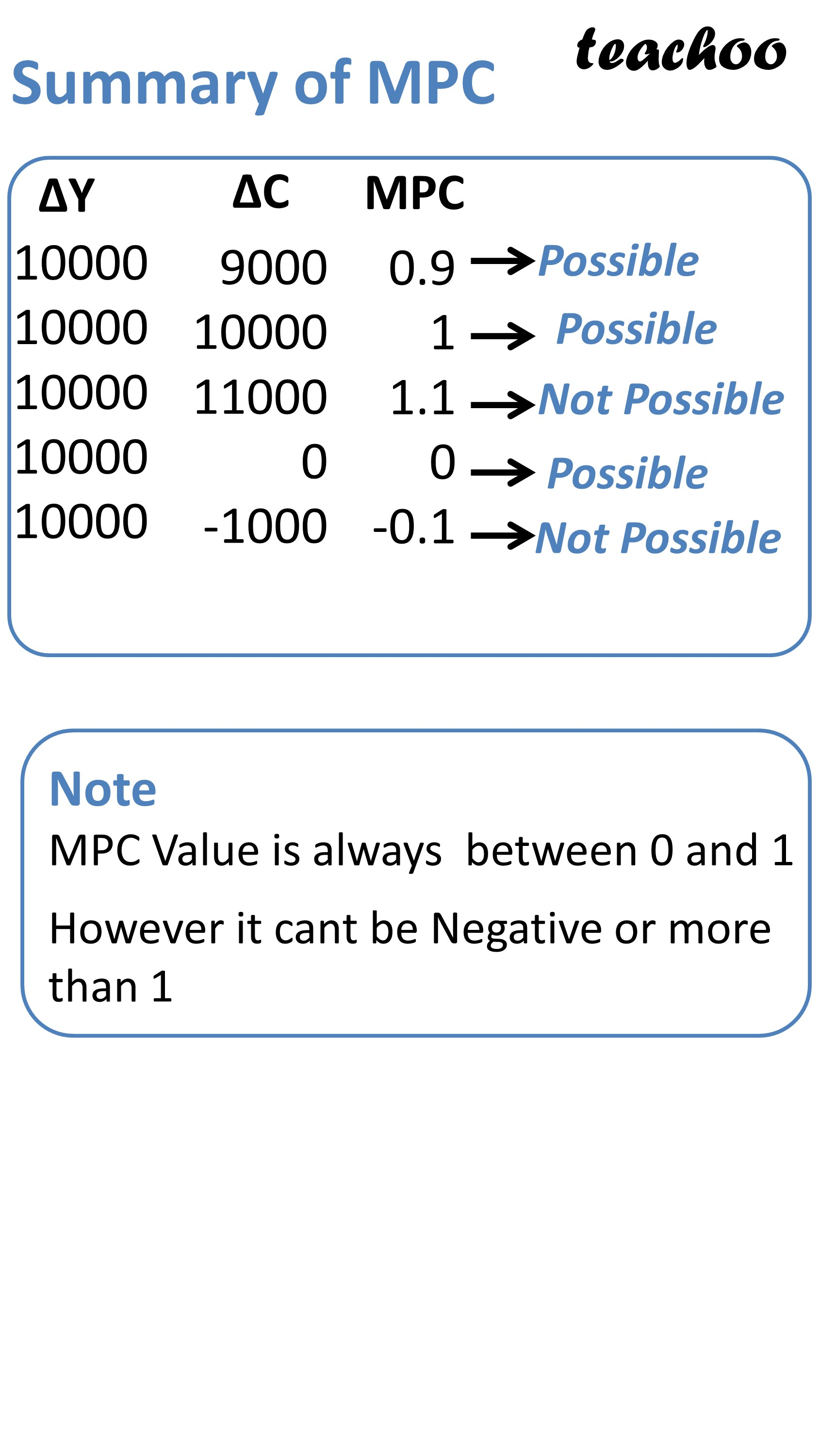Value of MPC is normally between 0 and 1

It remains same irrespective of level of income

## What happens when MPC is 0?

If MPC is 0, it means person is not spending additional income

## What happens when MPC = 1?

It means person is spending all of his additional income

## Why can't MPC be more than 1?

If MPC is more than 1, it means person is spending more than his additional income (which is not possible)

## Why cant MPC be negative?

If MPC is negative, it means persons income increased but he is spending even less than before

Difference between APC and MPC

 APC MPC It is the ratio of consumption expenditure to corresponding level of income at given point of time It is the ratio of change in consumption expenditure to corresponding change level of income APC = C/ Y MPC = Delta C/Delta Y It decreases with increase in income It remains constant and do not change with change in income It can be more than 1 or lower than 1 MPC is between 0 and 1 However it can't be 0 or negative It can't be negative

## How to Make Schedule and Graph of APC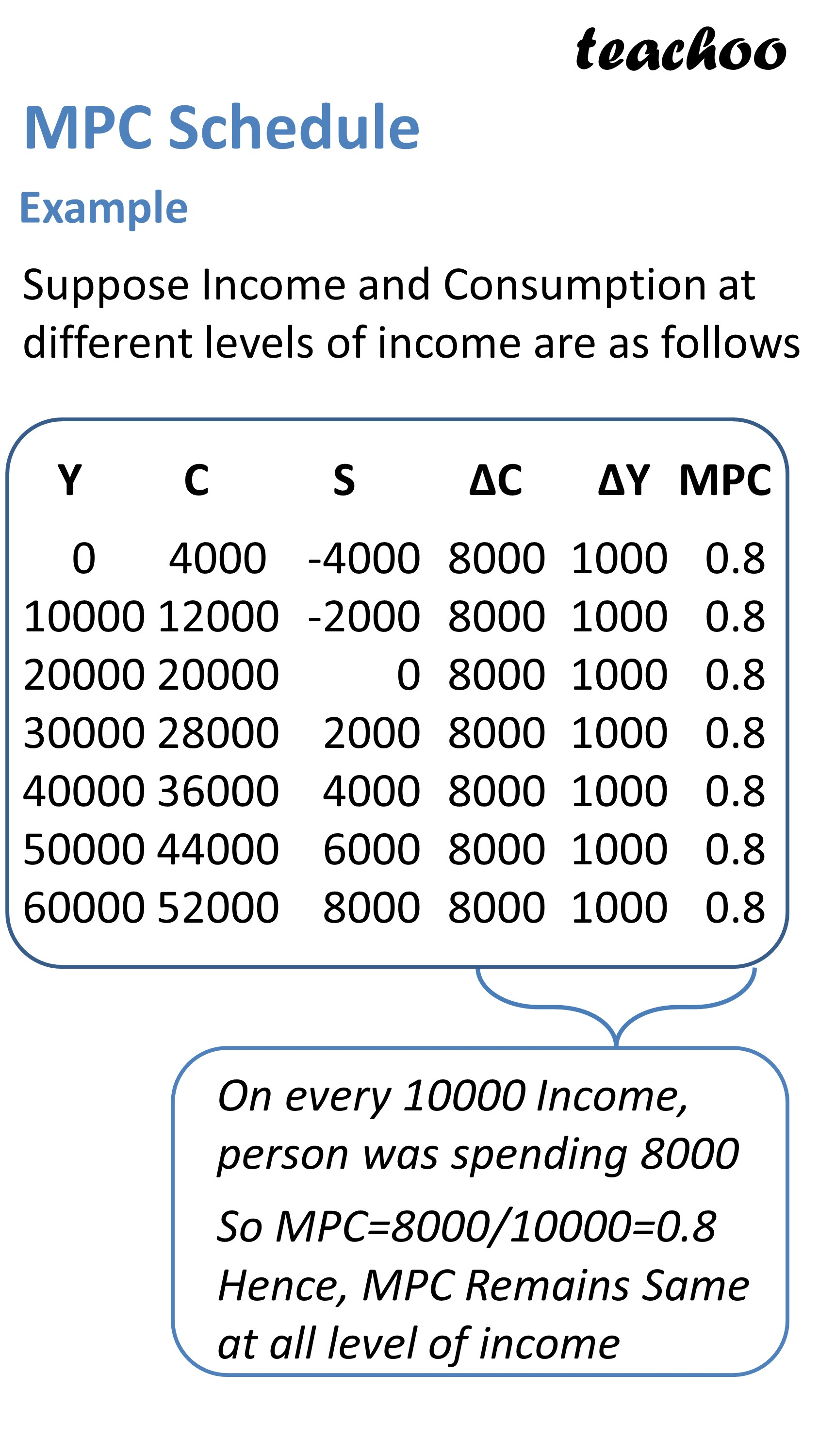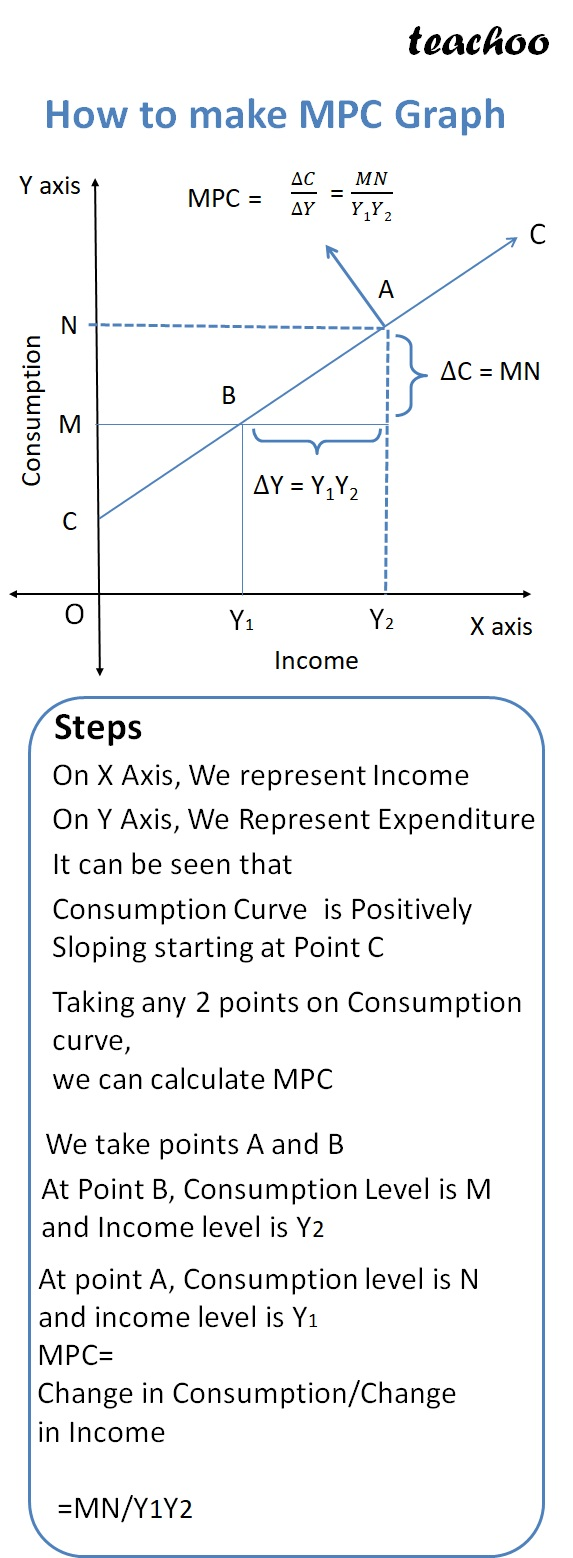### NCERT Questions

#### Question 1

What is marginal propensity to consume?

How is it related to marginal propensity to save?

### Other Books

#### Question 1

State the difference between APC and MPC.

Learn in your speed, with individual attention - Teachoo Maths 1-on-1 Class

### Transcript

How to Draw Graph of APS Steps On X Axis, We represent Income On Y Axis, We Represent Savings It can be seen that Savings Curve is Positively Sloping starting at Point S At any point on Savings Curve, we can calculate APS If we calculate at Point P, it is Savings/Income =OR/OY What is Marginal Propensity to Consume (MPC) Example 1 Suppose my Salary is Rs 50000 Out of this, I spend Rs 30000 My Salary Increased to Rs 60000 I now spend Rs 36000 Change in Consumption(ΔC) = 36000-30000=6000 Change in Income (ΔY) = 60000-50000=10000 Ratio of these 2 is MPC Marginal Propensity to Consume = Change in Consumption/Change in Income = ΔC/ΔY = 6000/10000 = 0.6 Does MPC Always Remain Same? Example Suppose Income and Consumption at different levels of income are as follows 0 10000 20000 30000 40000 50000 60000 4000 12000 20000 28000 36000 44000 52000 -4000 -2000 0 2000 4000 6000 8000 8000 8000 8000 8000 8000 8000 8000 1000 1000 1000 1000 1000 1000 1000 0.8 0.8 0.8 0.8 0.8 0.8 0.8 On every 10000 Income, person was spending 8000 So MPC=8000/10000=0.8 Hence, MPC Remains Same at all level of income What happens when MPC is 0 Example Suppose my Salary was Rs 50000 Out of this, I spent Rs 30000 My Salary Increased to Rs 60000 But I continue to spend Rs 30000 Change in Consumption (ΔC) =30000-30000 =0 Change in Income (ΔY) =60000-50000=10000 Marginal Propensity to Consume = Change in Consumption/Change in Income = 0/10000 = 0 Note If MPC is 0,it means person is not spending additional income What happens when MPC =1 Example Suppose my Salary was Rs 50000 Out of this, I spent Rs 30000 My Salary Increased to Rs 60000 But I continue to spend Rs 40000 Answer Change in Consumption (ΔC) 40000-30000 =10000 Change in Income (ΔY) 60000-50000=10000 Marginal Propensity to Consume = Change in Consumption/Change in Income = 10000/10000 = 1 Note If MPC is 1,it means person is spending all of his additional income Can MPC be more than 1? Example Suppose my Salary was Rs 50000 Out of this, I spent Rs 30000 My Salary Increased to Rs 60000 But I continue to spend Rs 42000 Answer Change in Consumption (ΔC) = 42000-30000 =12000 Change in Income (ΔY) = 60000-50000=10000 Marginal Propensity to Consume = ΔC /ΔY = 12000/10000 Note -If MPC is more than 1,it means person is spending more than his additional income . This is not possible as person can spend maximum up to amount of additional income earned and not more Can MPC be negative ? Suppose my Salary was Rs 50000 Out of this, I spent Rs 30000 Out of this, I spent Rs 30000 My Salary Increased to Rs 60000 But I now spend only Rs 29000 Answer Change in Consumption (ΔC) 29000-30000 =-1000 Change in Income (ΔY) = 60000-50000=10000 Marginal Propensity to Consume = ΔC /ΔY = -1000/10000 = -0.1 Note If MPC is less than 1,it means person is spending less on earning additional income .This is not possible because person expenditure always increases on earning additional income (and not decreases) Summary of MPC 10000 10000 10000 10000 10000 9000 10000 11000 0 -1000 0.9 1 1.1 0 -0.1 Note MPC Value is always between 0 and 1 However it cant be Negative or more than 1 MPC Schedule Example Suppose Income and Consumption at different levels of income are as follows 0 10000 20000 30000 40000 50000 60000 4000 12000 20000 28000 36000 44000 52000 -4000 -2000 0 2000 4000 6000 8000 8000 8000 8000 8000 8000 8000 8000 1000 1000 1000 1000 1000 1000 1000 0.8 0.8 0.8 0.8 0.8 0.8 0.8 On every 10000 Income, person was spending 8000 So MPC=8000/10000=0.8 Hence, MPC Remains Same at all level of income How to make MPC Graph Steps On X Axis, We represent Income On Y Axis, We Represent Expenditure It can be seen that Consumption Curve is Positively Sloping starting at Point C